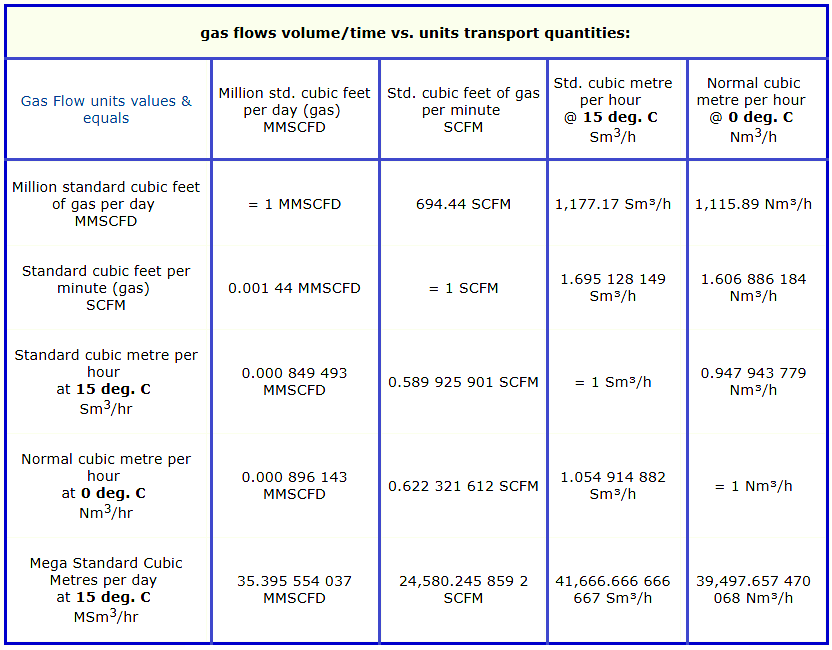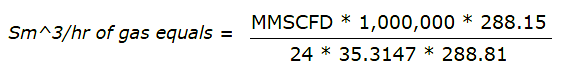# Gas Flow Formulas

### Gas Flow Units

MMSCFD – Million standard cubic feet of gas per day – a common volume of gas measure (unit abbreviation MMscf)
SCFD – Standard cubic feet per day (gas)
SCFM – Standard cubic feet per minute (gas)
Sm^3/hr – Standard cubic metre per hour defined at 15 deg. C – 59°F – for US
Nm^3/hr – Normal cubic metre per hour defined at zero deg. C – 32°F – for countries using SI metric system of units
MSm^3/d – Mega Standard Cubic Metres per day defined at 15 deg. Celsius – 59° Fahrenheit – outside of US

### Gas Flow Rate

Gas flow rate by cubic volume and motion velocity conversionsMath equations used to manually calculate gas flow outcomes

###Where:

288.15 = 15°C expressed in Kelvin (see below)

288.81 = 60°F expressed in Kelvin

24 = hours per day gas flow time

35.3147 = ft3 per m3

###Where:

273.15 = 0°C expressed in Kelvin (see below)

288.81 = 60°F expressed in Kelvin

24 = hours per day gas flow time

35.3147 = ft3 per m3

### Constants for the actual gas flow calculation

Volume ratio – 35.3147

0 degrees Celsius in K – 273.15

15 degrees Celsius in K – 288.15

59 degrees Fahrenheit in K – 288.81

hrs/day – 24

Ratio 60F/15°C – 0.997,714,761

Ratio 60F/0°C – 0.945,777,501

Please note that some numbers in these mathematical examples are rounded.

### Calculation Explanation :

The S and N letter obviously refers to the gas condition (standard and normal). Both conditions are at atmospheric pressure (1 atm or 101.325 kPa) with standard referring to 15ºC and normal to 0ºC gas temperature.

Firstly when comparing a gas at the same pressures and at different temperatures, the density increases as the temperature decreases. Therefore a certain mass of gas will have a smaller volume at normal conditions as apposed to standard conditions.

Now, 1 ft3 = 0.0283168 m3, 1 sft3 = 0.0283168 sm3 and 1 nft3 = 0.0283168 nm3. So, lets look at an example:

A gas well produces natural gas at a flow rate of 140 000 SCFD (standard cubic feet per day) at a stable state:

140 000 SCFD = 3964.352 sm3/day = 165.18 sm3/hr (this online gas flow converter gives very accurate sm3/hr readings, and for other gas transfer units it contains – without rounding errors.)

Now, using the equation PV=nRT for the both states, knowing that the P, n and R remains constant the equation can be written as follows:

V1 / V2 = T1 / T2
V1 = V2 x T1 / T2

Assuming condition 1 is at standard conditions (15ºC, 288.15K), and condition 2 is at normal conditions (0ºC, 273.15K), the gas volume based flow rate can be converted as follows:

165.18 = V2 x (288.15 / 273.15)
V2 = 156.58 nm3/hr (gives great nm3/h which is less < than the sm3/h)

1.